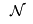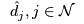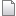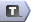# The Transshipment Problem in AMPL

The formulation of the transshipment problem in AMPL we present here is a straightforward translation of the alternative mathematical programme for the transshipment problem. We will build the file transshipment.mod.

The setis declared as NODES:

set NODES;


The net demandis declared as an integer parameter (note there is no >= 0):

param NetDemand {NODES} integer, default 0;

Setting the default NetDemand to be 0 means that no values need to be entered for transshipment nodes.

Note that the set NODES and the parameter NetDemand can be easily created from SUPPLY_NODES, DEMAND_NODES, Supply, etc, e.g.,:

set SUPPLY_NODES;
set DEMAND_NODES;
set TRANSSHIPMENT_NODES;

set NODES := SUPPLY_NODES union
TRANSSHIPMENT_NODES union
DEMAND_NODES;

param Supply {SUPPLY_NODES} >= 0, integer;
param Demand {DEMAND_NODES} >= 0, integer;

param NetDemand {n in NODES} integer
:= if n in SUPPLY_NODES then -Supply[n]
else if n in DEMAND_NODES then Demand[n]; # else 0 by default

Note that no default value is needed for NetDemand as it is explicitly defined for all nodes.

The set ARCS is defined between pairs of nodes and costs and bounds are also defined:

set ARCS within NODES cross NODES;

param Cost {ARCS};

param Lower {ARCS} >= 0, integer, default 0;
param Upper {(i, j) in ARCS} >= Lower[i, j], integer, default Infinity;


Now, the mathematical programme follows directly:

var Flow {(i, j) in ARCS} >= Lower[i, j], <= Upper[i, j], integer;

minimize TotalCost:
sum {(i, j) in ARCS} Cost[i, j] * Flow[i, j];

subject to ConserveFlow {j in NODES}:
sum {(i, j) in ARCS} Flow[i, j] - sum {(j, k) in ARCS} Flow[j, k] >= NetDemand[j];


-- MichaelOSullivan - 06 Oct 2014

Topic attachments
I Attachment History Action Size Date Who Commentmod transshipment.mod r5 r4 r3 r2 r1 manage 1.5 K 2008-04-28 - 02:21 MichaelOSullivan
Topic revision: r8 - 2019-11-11 - MichaelOSullivanCopyright © 2008-2023 by the contributing authors. All material on this collaboration platform is the property of the contributing authors.
Ideas, requests, problems regarding TWiki? Send feedback